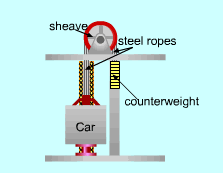Ch 3. First Law of Thermodynamics Multimedia Engineering Thermodynamics Conservationof Mass Conservationof Energy Solids andLiquids Ideal Gas
 Chapter 1. Basics 2. Pure Substances 3. First Law 4. Energy Analysis 5. Second Law 6. Entropy 7. Exergy Analysis 8. Gas Power Cyc 9. Brayton Cycle 10. Rankine Cycle Appendix Basic Math Units Thermo Tables Search eBooks Dynamics Fluids Math Mechanics Statics Thermodynamics Author(s): Meirong Huang Kurt Gramoll ©Kurt GramollTHERMODYNAMICS - CASE STUDY IntroductionHow the Elevator Works Noel is an electric engineer who is designing an elevator for the new wing of the library. The elevator needs to carry new books to the fourth floor where the university bookstore is located. The elevator is always fully loaded when it travels upwards and empty when it travels downwards. The main power line was installed when the library was built, hence the power for this elevator is limited. Noel needs to make sure his design is feasible for the power requirement. What is known: The mass of the empty car mcar = 150 kg The maximum load is mload = 650 kg The mass of the counterweight is 50% of the total mass of the elevator when it is fully loaded The operating velocity of the elevator is v = 2.0 m/s Questions Determine the power required when the elevator is fully loaded and moving upwards Determine the power required when the elevator is empty and moving downwards How much energy can be saved by using counterweight for a single delivery? Approach Consider the car and the counterweight together as a system Consider the energy change for a time span from t1 to t2